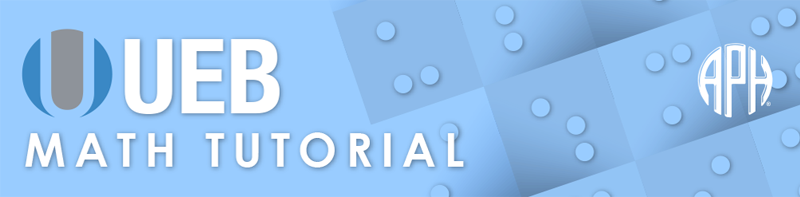Practice Problems -   -  Use 6 Dot Entry   Switch to Nemeth Tutorial

# Lesson 10.3: Logic Notation

## Symbols

$\vee \phantom{\rule{.3em}{0ex}}\text{or (upright v shape)}$
⠈⠖

$\wedge \phantom{\rule{.3em}{0ex}}\text{and (inverted v shape)}$
⠈⠦

$¬\phantom{\rule{.3em}{0ex}}\text{not}$
⠈⠹

$\forall \phantom{\rule{.3em}{0ex}}\text{for or (inverted A)}$
⠘⠁

$\exists \phantom{\rule{.3em}{0ex}}\text{there exists (reverse E)}$
⠘⠢

$\therefore \phantom{\rule{.3em}{0ex}}\text{therefore}$
⠠⠡

$\equiv \phantom{\rule{.3em}{0ex}}\text{identical to}$
⠸⠿

$\oplus \phantom{\rule{.3em}{0ex}}\text{circle enclosing plus sign}$
⠰⠫⠿⠪⠐⠖

$⊻\phantom{\rule{.3em}{0ex}}\text{v bar}$
⠈⠖⠠⠱

## Review

A general principle of UEB is that each print symbol has one and only one braille equivalent. UEB follows this principle by using the same physical symbol regardless of context (literary or technical). Punctuation symbols that appear within mathematical expressions in print are the same physical symbols used within literary contexts.

There are symbols that have a grade 2 meaning therefore they must be in grade 1 mode to ensure that the symbol is not confused with the contracted meaning.

## Explanation

There are several symbols used in logic and set theory that have not been introduced in previous lessons. A complete listing may be found in Guidelines for Technical Material 2014 §10 and §11. A few of the most commonly used logic notation symbols are presented in this lesson. It must be noted that symbols may have multiple meanings and or names depending on the related field of mathematics in which they are used. The following basic symbols will be referred to in terms of how they are spoken. For example, the symbol for negation is read as "not".

The "not" symbol is a horizontal line with a short line extending downward from the right side. The "not" symbol in braille is dot 1, dots one four five six. The tilde is also used in print for "not" notation.

### Example 1

$¬p$
⠈⠹⠏

### Example 2

$~p$
⠈⠔⠏

The "and" symbol is a connective and looks like an inverted V in print. This is a two cell symbol that is brailled with dot four in the first cell and dots two three six in the second cell. The ampersand is also used in print for the "and" notation.

### Example 3

$p\wedge q$
⠏⠈⠦⠟

### Example 4

$p&q$
⠏⠈⠯⠟

The "or" symbol is a connective and looks like an upper-case letter V. It is brailled as dot four, dots two three six.

### Example 5

$p\vee q$
⠏⠈⠖⠟

The "if then" symbol is a right arrow. The following example is read "if p then q".

### Example 6

$p\to q$
⠰⠰⠰⠏⠀⠳⠕⠀⠟⠰⠄

The "therefore" symbol in print is three dots shown in an upright triangle formation. It is brailled as dot six, dots one six.

### Example 7

$p\therefore q$
⠰⠰⠰⠏⠀⠠⠡⠀⠟⠰⠄

The "if and only if" symbol is most commonly stated by a two-way arrow.

### Example 8

$p↔q$
⠰⠰⠰⠏⠀⠳⠺⠗⠕⠀⠟⠰⠄

The "identical to" symbol is an equal sign with a horizontal line above in print. It is brailled as dots four five six, dots one two three four five six.

### Example 9

$p\equiv q$
⠰⠰⠰⠏⠀⠸⠿⠀⠟⠰⠄

The "either or but not both" symbol is commonly the XOR. This is a circle shape with a plus sign shown inside of it. The braille symbol for physical enclosure, dots two four six, immediately follows the circle and precedes the plus sign. The entire symbol is brailled using 5 cells, dots one two four six, dots one two three four five six, dots two four six, dot five, and dots two three five. The shape termination indicator must be used unless it is immediately followed by a space.

### Example 10

$p\oplus q$
⠰⠰⠏⠫⠿⠪⠐⠖⠱⠟

Another "either or but not both" symbol is the V bar. This is the or symbol with a bar directly below it in print. This symbol is brailled as the "or" symbol followed by the bar under previous item indicator. It uses four cells: dot four, dots two three five, dot six, dots one five six.

### Example 11

$p⊻q$
⠰⠰⠏⠈⠖⠠⠱⠟

The "for all" symbol is an inverted upper-case letter A in print. It is brailled as dots four five, dot one.

### Example 12

$\forall x$
⠘⠁⠭

The "there exists" symbol looks like a reversed upper-case letter E in print. It is brailled as dots four five, dots two six.

### Example 13

$\exists x$
⠘⠢⠭